## VI. LABORATORY ACTIVITY 1. Look for a publicly available data set with at least 50 rows that will...

Regression analysis using R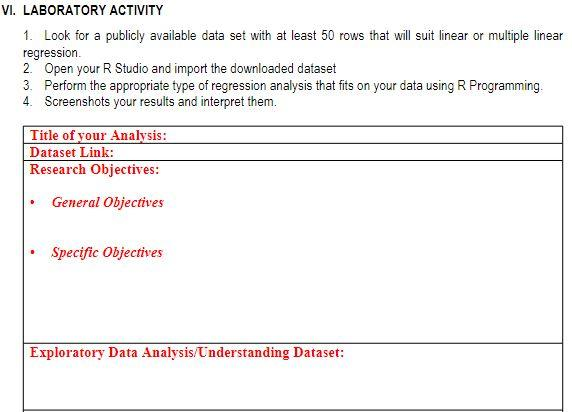Solved
COMPUTER SCIENCE 1 Answer Noor Mohammed

## Quia Name: ID: Sec: Instruction: Complete the thesis statement (1.5 marks), write topic sentences...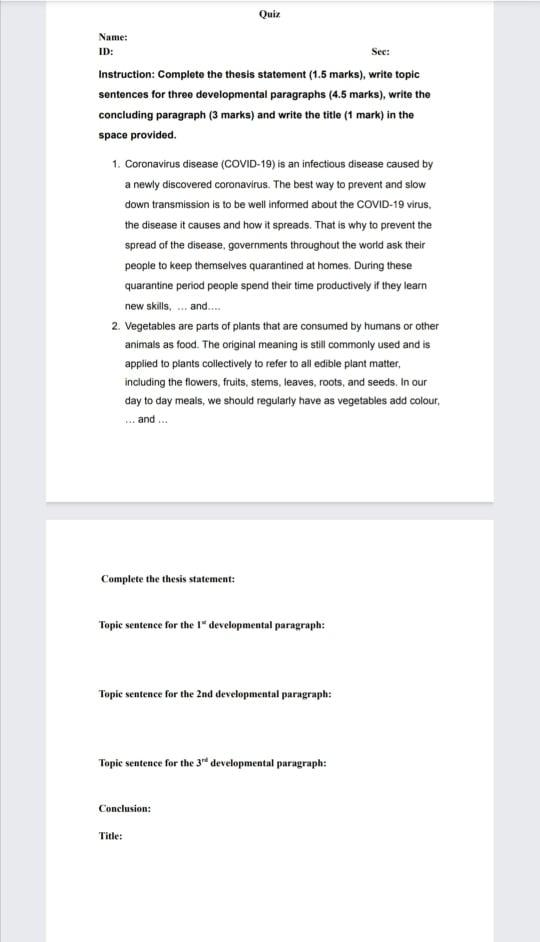Solved
ECONOMICS 1 Answer Loren Hannah Coronel

## Write a class studentInfo that represents a student record. It must have variables to store the s...

write a CPP program using template class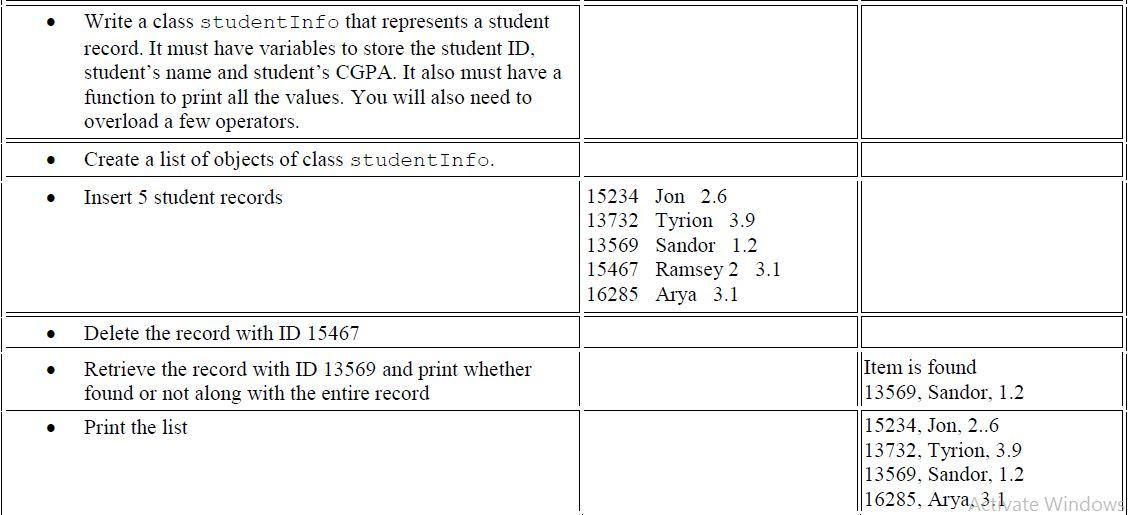Solved
COMPUTER SCIENCE 1 Answer rahul verma

## 2. The table below summarizes information about the market for the principles of economics textbo...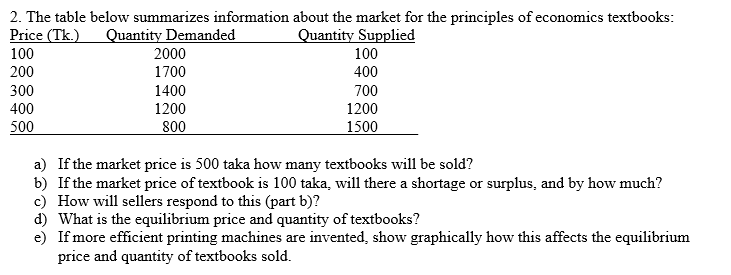Solved

## a) The diagrams shown below are the result of executing an algorithm which has one paramet...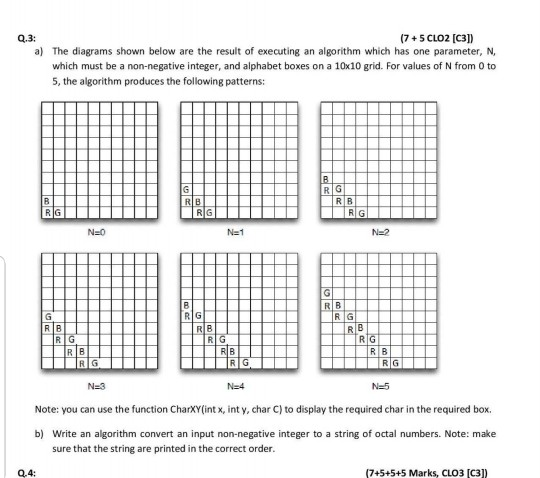a) The diagrams shown below are the result of executing an algorithm which has one parameter, N, which must be a non-negative integer, and alphabet boxes on a 10<10 grid. For values of N from O to 5, the algorithm produces the following patterns:

Solved
COMPUTER SCIENCE 1 Answer Hanzel Paz

## A blocked rotor test was performed on the motor of the following were obtained line to line volta...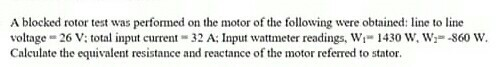Solved
Electrical Engineering 1 Answer halfblood Prince

## A blocked rotor test was performed on the motor of the following were obtained line to line volta...Solved
Electrical Engineering 1 Answer Ammar Wafiy Rosli

## Calculate the equivalent resistance and reactance of the motor referred to stator.

The blocked-rotor test was performed on the motor of the following were obtained: line to line voltage = 26 V; total input current = 32 A; input wattmeter readings, W_1 = 1430 W; W_2 = -860 W. Calculate the equivalent resistance and reactance of the motor referred to stator.

in progress
Electrical Machines 1 Answer Sam Sumalinog

## Calculate the equivalent resistance and reactance of the motor referred to stator.

The blocked-rotor test was performed on the motor of the following were obtained: line to line voltage = 26 V; total input current = 32 A; input wattmeter readings, W_1 = 1430 W; W_2 = -860 W. Calculate the equivalent resistance and reactance of the motor referred to stator.

in progress
Electrical Machines 1 Answer Sam Sumalinog

## Coal-burning electrical power station. Large central power stations...

Coal-burning electrical power station. Large central power stations (about 1000 MW electrical) using fluidized bed combustors may be built someday
(see Fig. P1.2). These giants would be fed 240 tons of collars (90% C, 10%H,), 50% of which would burn within the battery of primary fluidized beds,
the other 50% elsewhere in the system. One suggested design would use a battery of 10 fluidized beds, each 20 m long, 4 m wide, and containing solids
to a depth of 1 m. Find the rate of reaction within the beds, based on the oxygen used.

in progress
Chemical Engineering 1 Answer Büşra EMİR